# Selina solutions for Concise Mathematics Class 8 ICSE chapter 20 - Area of a Trapezium and a Polygon [Latest edition]

#### Chapters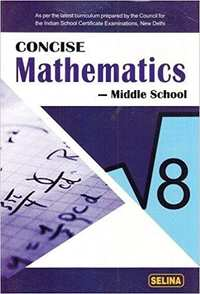## Chapter 20: Area of a Trapezium and a Polygon

Exercise 20 (A)Exercise 20 (B)Exercise 20 (C)Exercise 20 (D)
Exercise 20 (A) [Pages 223 - 224]

### Selina solutions for Concise Mathematics Class 8 ICSE Chapter 20 Area of a Trapezium and a PolygonExercise 20 (A) [Pages 223 - 224]

Exercise 20 (A) | Q 1.1 | Page 223

Find the area of a triangle, whose sides are :

10 cm, 24 cm, and 26 cm

Exercise 20 (A) | Q 1.2 | Page 223

Find the area of a triangle, whose sides are :

18 mm, 24 mm and 30 mm

Exercise 20 (A) | Q 1.3 | Page 223

Find the area of a triangle, whose sides are :

21 m, 28 m, and 35 m

Exercise 20 (A) | Q 2 | Page 223

Two sides of a triangle are 6 cm and 8 cm. If the height of the triangle corresponding to 6 cm side is 4 cm; find :

(i) area of the triangle
(ii) height of the triangle corresponding to 8 cm side.

Exercise 20 (A) | Q 3 | Page 223

The sides of a triangle are 16 cm, 12 cm, and 20 cm. Find :

(i) area of the triangle ;
(ii) height of the triangle, corresponding to the largest side ;
(iii) height of the triangle, corresponding to the smallest side.

Exercise 20 (A) | Q 4 | Page 224

Two sides of a triangle are 6.4 m and 4.8 m. If the height of the triangle corresponding to 4.8 m side is 6 m;

find :
(i) area of the triangle ;
(ii) height of the triangle corresponding to 6.4 m sides.

Exercise 20 (A) | Q 5 | Page 224

The base and the height of a triangle are in the ratio 4: 5. If the area of the triangle is 40 m2; find its base and height.

Exercise 20 (A) | Q 6 | Page 224

The base and the height of a triangle are in the ratio 5 : 3. If the area of the triangle is 67.5 m2; find its base and height.

Exercise 20 (A) | Q 7 | Page 224

The area of an equilateral triangle is 144sqrt3 cm2; find its perimeter.

Exercise 20 (A) | Q 8 | Page 224

The area of an equilateral triangle is numerically equal to its perimeter. Find its perimeter correct to 2 decimal places.

Exercise 20 (A) | Q 9 | Page 224

A field is in the shape of a quadrilateral ABCD in which side AB = 18 m, side AD = 24 m, side BC = 40m, DC = 50 m and angle A = 90°. Find the area of the field.

Exercise 20 (A) | Q 10 | Page 224

The lengths of the sides of a triangle are in the ratio 4: 5 : 3 and its perimeter is 96 cm. Find its area.

Exercise 20 (A) | Q 11 | Page 224

One of the equal sides of an isosceles triangle is 13 cm and its perimeter is 50 cm. Find the area of the triangle.

Exercise 20 (A) | Q 12 | Page 224

The altitude and the base of a triangular field are in the ratio 6: 5. If its cost is ₹ 49,57,200 at the rate of ₹ 36,720 per hectare and 1 hectare = 10,000 sq. m, find (in metre) dimensions of the field.

Exercise 20 (A) | Q 13 | Page 224

Find the area of the right-angled triangle with hypotenuse 40 cm and one of the other two sides 24 cm.

Exercise 20 (A) | Q 14 | Page 224

Use the information given in the adjoining figure to find :
(i) the length of AC.
(ii) the area of an ∆ABC
(iii) the length of BD, correct to one decimal place.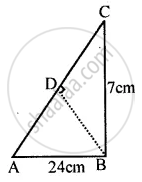Exercise 20 (B) [Pages 227 - 228]

### Selina solutions for Concise Mathematics Class 8 ICSE Chapter 20 Area of a Trapezium and a PolygonExercise 20 (B) [Pages 227 - 228]

Exercise 20 (B) | Q 1 | Page 227

Find the length and perimeter of a rectangle, whose area = 120 cm2 and breadth = 8 cm

Exercise 20 (B) | Q 2 | Page 227

The perimeter of a rectangle is 46 m and its length is 15 m. Find its :
(ii) area
(iii) diagonal.

Exercise 20 (B) | Q 3 | Page 227

The diagonal of a rectangle is 34 cm. If its breadth is 16 cm; find its :
(i) length
(ii) area

Exercise 20 (B) | Q 4 | Page 227

The area of a small rectangular plot is 84 m2. If the difference between its length and the breadth is 5 m; find its perimeter.

Exercise 20 (B) | Q 5 | Page 227

The perimeter of a square is 36 cm; find its area

Exercise 20 (B) | Q 6 | Page 227

Find the perimeter of a square; whose area is: 1.69 m2

Exercise 20 (B) | Q 7 | Page 227

The diagonal of a square is 12 cm long; find its area and length of one side.

Exercise 20 (B) | Q 8 | Page 227

The diagonal of a square is 15 m; find the length of its one side and perimeter.

Exercise 20 (B) | Q 9 | Page 227

The area of a square is 169 cm2. Find its:
(i) one side
(ii) perimeter

Exercise 20 (B) | Q 10 | Page 227

The length of a rectangle is 16 cm and its perimeter is equal to the perimeter of a square with side 12.5 cm. Find the area of the rectangle.

Exercise 20 (B) | Q 11 | Page 227

The perimeter of a square is numerically equal to its area. Find its area.

Exercise 20 (B) | Q 12 | Page 227

Each side of a rectangle is doubled. Find the ratio between :
(i) perimeters of the original rectangle and the resulting rectangle.
(ii) areas of the original rectangle and the resulting rectangle.

Exercise 20 (B) | Q 13 | Page 227

In each of the following cases, ABCD is a square and PQRS is a rectangle. Find, in each case, the area of the shaded portion.
(All measurements are in metre).

(i)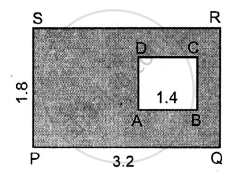(ii)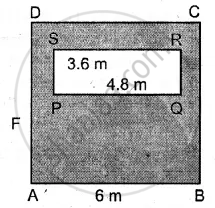Exercise 20 (B) | Q 14 | Page 227

A path of uniform width, 3 m, runs around the outside of a square field of side 21 m. Find the area of the path.

Exercise 20 (B) | Q 15 | Page 227

A path of uniform width, 2.5 m, runs around the inside of a rectangular field 30 m by 27 m. Find the area of the path.

Exercise 20 (B) | Q 16 | Page 227

The length of a hall is 18 m and its width is 13.5 m. Find the least number of square tiles, each of side 25 cm, required to cover the floor of the hall,

(i) without leaving any margin.
(ii) leaving a margin of width 1.5 m all around. In each case, find the cost of the tiles required at the rate of Rs. 6 per tile

Exercise 20 (B) | Q 17 | Page 227

A rectangular field is 30 m in length and 22m in width. Two mutually perpendicular roads, each 2.5 m wide, are drawn inside the field so that one road is parallel to the length of the field and the other road is parallel to its width. Calculate the area of the crossroads.

Exercise 20 (B) | Q 18 | Page 228

The length and the breadth of a rectangular field are in the ratio 5: 4 and its area is 3380 m2. Find the cost of fencing it at the rate of ₹75 per m.

Exercise 20 (B) | Q 19 | Page 228

The length and the breadth of a conference hall are in the ratio 7: 4 and its perimeter is 110 m. Find:

(i) area of the floor of the hall.
(ii) a number of tiles, each a rectangle of size 25 cm x 20 cm, required for the flooring of the hall.
(iii) the cost of the tiles at the rate of ₹ 1,400 per hundred tiles.

Exercise 20 (C) [Page 232]

### Selina solutions for Concise Mathematics Class 8 ICSE Chapter 20 Area of a Trapezium and a PolygonExercise 20 (C) [Page 232]

Exercise 20 (C) | Q 1 | Page 232

The following figure shows the cross-section ABCD of a swimming pool which is a trapezium in shape.
If the width DC, of the swimming pool, is 6.4cm, depth (AD) at the shallow end is 80 cm and depth (BC) at the deepest end is 2.4m, find Its area of the cross-section.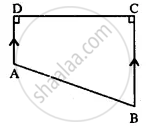Exercise 20 (C) | Q 2 | Page 232

The parallel sides of a trapezium are in ratio 3: 4. If the distance between the parallel sides is 9 dm and its area is 126 dm2; find the lengths of its parallel sides.

Exercise 20 (C) | Q 3 | Page 232

The two parallel sides and the distance between them are in the ratio 3: 4: 2. If the area of the trapezium is 175 cm2, find its height.

Exercise 20 (C) | Q 4 | Page 232

A parallelogram has sides of 15 cm and 12 cm; if the distance between the 15 cm sides is 6 cm; find the distance between 12 cm sides.

Exercise 20 (C) | Q 5 | Page 232

A parallelogram has sides of 20 cm and 30 cm. If the distance between its shorter sides is 15 cm; find the distance between the longer sides.

Exercise 20 (C) | Q 6 | Page 232

The adjacent sides of a parallelogram are 21 cm and 28 cm. If it's one diagonal is 35 cm; find the area of the parallelogram.

Exercise 20 (C) | Q 7 | Page 232

The diagonals of a rhombus are 18 cm and 24 cm. Find:
(i) its area ;
(ii) length of its sides.
(iii) its perimeter

Exercise 20 (C) | Q 8 | Page 232

The perimeter of a rhombus is 40 cm. If one diagonal is 16 cm; find :
(i) it's another diagonal

Exercise 20 (C) | Q 9 | Page 232

Each side of a rhombus is 18 cm. If the distance between two parallel sides is 12 cm, find its area.

Exercise 20 (C) | Q 10 | Page 232

The length of the diagonals of a rhombus is in ratio 4 : 3. If its area is 384 cm2, find its side.

Exercise 20 (C) | Q 11 | Page 232

A thin metal iron-sheet is rhombus in shape, with each side 10 m. If one of its diagonals is 16 m, find the cost of painting both sides at the rate of ₹ 6 per m2. Also, find the distance between the opposite sides of this rhombus.

Exercise 20 (C) | Q 12 | Page 232

The area of a trapezium is 279 sq.cm and the distance between its two parallel sides is 18 cm. If one of its parallel sides is longer than the other side by 5 cm, find the lengths of its parallel sides.

Exercise 20 (C) | Q 13 | Page 232

The area of a rhombus is equal to the area of a triangle. If the base ∆ is 24 cm, its corresponding altitude is 16 cm and one of the diagonals of the rhombus is 19.2 cm. Find its other diagonal.

Exercise 20 (C) | Q 14 | Page 232

Find the area of the trapezium ABCD in which AB || DC, AB = 18 cm, ∠B = ∠C = 90°, CD = 12 cm and AD = 10 cm.

Exercise 20 (D) [Pages 234 - 235]

### Selina solutions for Concise Mathematics Class 8 ICSE Chapter 20 Area of a Trapezium and a PolygonExercise 20 (D) [Pages 234 - 235]

Exercise 20 (D) | Q 1 | Page 234

Find the radius and area of a circle, whose circumference is :
(i) 132 cm
(ii) 22 m

Exercise 20 (D) | Q 2 | Page 234

Find the radius and circumference of a circle, whose area is :
(i) 154 cm2
(ii) 6.16 m2

Exercise 20 (D) | Q 3 | Page 234

The circumference of a circular table is 88 m. Find its area.

Exercise 20 (D) | Q 4 | Page 234

The area of a circle is 1386 sq.cm; find its circumference.

Exercise 20 (D) | Q 5 | Page 234

Find the area of a flat circular ring formed by two concentric circles (circles with the same centre) whose radii are 9 cm and 5 cm.

Exercise 20 (D) | Q 6 | Page 234

Find the area of the shaded portion in each of the following diagrams :

(i)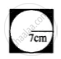(ii)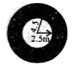Exercise 20 (D) | Q 7 | Page 234

The radii of the inner and outer circumferences of a circular running track are 63 m and 70 m respectively. Find :
(i) the area of the track ;
(ii) the difference between the lengths of the two circumferences of the track.

Exercise 20 (D) | Q 8 | Page 234

A circular field of radius 105 m has a circular path of uniform width of 5 m along and inside its boundary. Find the area of the path.

Exercise 20 (D) | Q 9 | Page 234

There is a path of uniform width 7 m round and outside a circular garden of diameter 210 m. Find the area of the path.

Exercise 20 (D) | Q 10 | Page 234

A wire, when bent in the form of a square encloses an area of 484 cm2. Find :
(i) one side of the square ;
(ii) length of the wire ;
(iii) the largest area enclosed; if the same wire is bent to form a circle.

Exercise 20 (D) | Q 11 | Page 234

A wire, when bent in the form of a square; encloses an area of 196 cm2. If the same wire is bent to form a circle; find the area of the circle.

Exercise 20 (D) | Q 12 | Page 235

The radius of a circular wheel is 42 cm. Find the distance travelled by it in :
(i) 1 revolution ;
(ii) 50 revolutions ;
(iii) 200 revolutions ;

Exercise 20 (D) | Q 13 | Page 235

The diameter of a wheel is 0.70 m. Find the distance covered by it in 500 revolutions. If the wheel takes 5 minutes to make 500 revolutions; find its speed in :
(i) m/s
(ii) km/hr.

Exercise 20 (D) | Q 14 | Page 235

A bicycle wheel, diameter 56 cm, is making 45 revolutions in every 10 seconds. At what speed in kilometre per hour is the bicycle traveling?

Exercise 20 (D) | Q 15 | Page 235

A roller has a diameter of 1.4 m. Find :
(i) its circumference ;
(ii) the number of revolutions it makes while travelling 61.6 m.

Exercise 20 (D) | Q 16 | Page 235

Find the area of the circle, length of whose circumference is equal to the sum of the lengths of the circumferences with radii 15 cm and 13 cm.

Exercise 20 (D) | Q 17 | Page 235

A piece of wire of length 108 cm is bent to form a semicircular arc bounded by its diameter. Find its radius and area enclosed.

Exercise 20 (D) | Q 18 | Page 235

In the following figure, a rectangle ABCD enclosed three circles. If BC = 14 cm, find the area of the shaded portion (Take π = 22/7)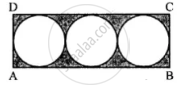## Chapter 20: Area of a Trapezium and a Polygon

Exercise 20 (A)Exercise 20 (B)Exercise 20 (C)Exercise 20 (D)## Selina solutions for Concise Mathematics Class 8 ICSE chapter 20 - Area of a Trapezium and a Polygon

Selina solutions for Concise Mathematics Class 8 ICSE chapter 20 (Area of a Trapezium and a Polygon) include all questions with solution and detail explanation. This will clear students doubts about any question and improve application skills while preparing for board exams. The detailed, step-by-step solutions will help you understand the concepts better and clear your confusions, if any. Shaalaa.com has the CISCE Concise Mathematics Class 8 ICSE solutions in a manner that help students grasp basic concepts better and faster.

Further, we at Shaalaa.com provide such solutions so that students can prepare for written exams. Selina textbook solutions can be a core help for self-study and acts as a perfect self-help guidance for students.

Concepts covered in Concise Mathematics Class 8 ICSE chapter 20 Area of a Trapezium and a Polygon are Perimeter of Triangles, Perimeter of Squares, Area of a Parallelogram, Area of a Rhombus, Area of Circle, Area of Trapezium, Perimeter of a Rectangle.

Using Selina Class 8 solutions Area of a Trapezium and a Polygon exercise by students are an easy way to prepare for the exams, as they involve solutions arranged chapter-wise also page wise. The questions involved in Selina Solutions are important questions that can be asked in the final exam. Maximum students of CISCE Class 8 prefer Selina Textbook Solutions to score more in exam.

Get the free view of chapter 20 Area of a Trapezium and a Polygon Class 8 extra questions for Concise Mathematics Class 8 ICSE and can use Shaalaa.com to keep it handy for your exam preparation